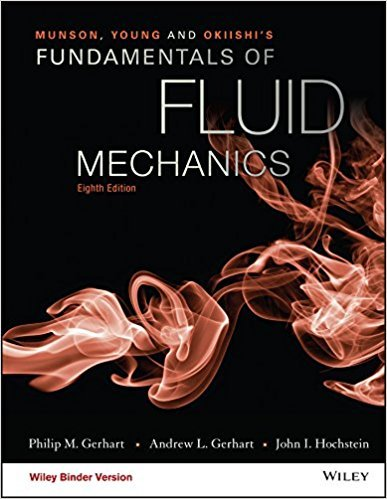×
Get Full Access to Fundamentals Of Fluid Mechanics - 8 Edition - Chapter 8.4.1. - Problem 8.46
Get Full Access to Fundamentals Of Fluid Mechanics - 8 Edition - Chapter 8.4.1. - Problem 8.46

×

# Von Karman suggested that the wholly turbulent friction factorbe expressed by theISBN: 9781119080701 456

## Solution for problem 8.46 Chapter 8.4.1.

Fundamentals of Fluid Mechanics | 8th Edition

• Textbook Solutions
• 2901 Step-by-step solutions solved by professors and subject experts
• Get 24/7 help from StudySoup virtual teaching assistantsFundamentals of Fluid Mechanics | 8th Edition

4 5 1 254 Reviews
17
0
Problem 8.46

Von Karman suggested that the wholly turbulent friction factorbe expressed by the equationf = 14[0.57 log(D)]2,where is the absolute roughness of the pipe. Compare the valuespredicted by this equation and those indicated on the Moody chart.

Step-by-Step Solution:
Step 1 of 3

COP2271 1/10/17 Programming language- particular set of rules/instructions that tells your computer what to do CPU- central processing unit (brains of computer)  machine code-set of numeric code programmed into the CPU (I3, I5, I7) o 01011101  binary- 0, 1 (base 2)  hex- 0-9, A-F (base 16) MEMORY- RAM, L cache, hard drive (digital scratch paper) Program languages: MATLAB, C++ (c), Ruby, Java, Fortran, Python, C#, google go Compiled languages vs. Interpreted | C++ | MATLAB Separate program called a complier MATLAB software read your code 1 line at a that turns text-based code to machine code. time and tells the CPU what to do. (easier to use but slower). *MATLAB uses the .m extension (Hwl.m) (i.e. .doc, .xls) *Notes(comments)- % sign in front of the note (has to be before or after a complete line of code) Structure of the MATLAB script - % Note about the program - clc; clear;

Step 2 of 3

Step 3 of 3

## Discover and learn what students are asking

Chemistry: The Central Science : Introduction:Matter, Energy, and Measurement
?Which of the following diagrams represents a chemical change? [Section 1.3]

Unlock Textbook Solution